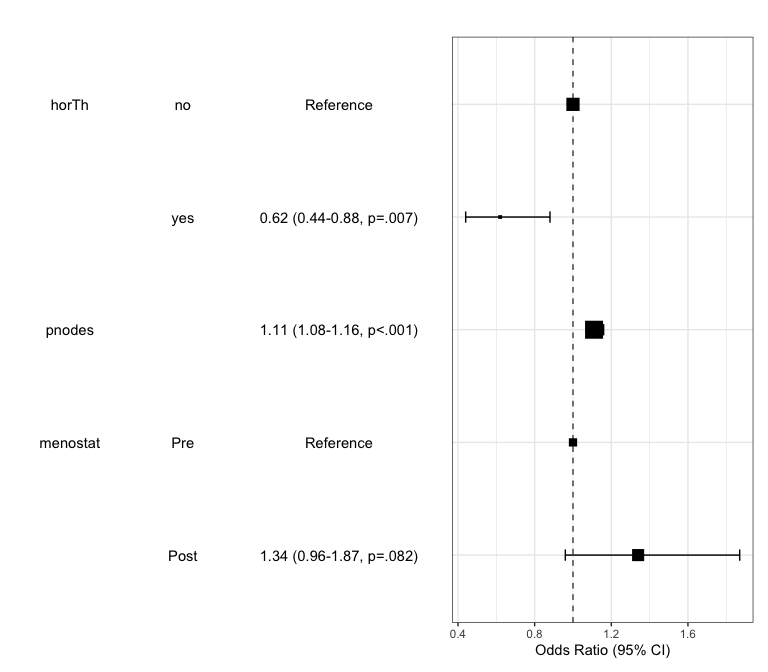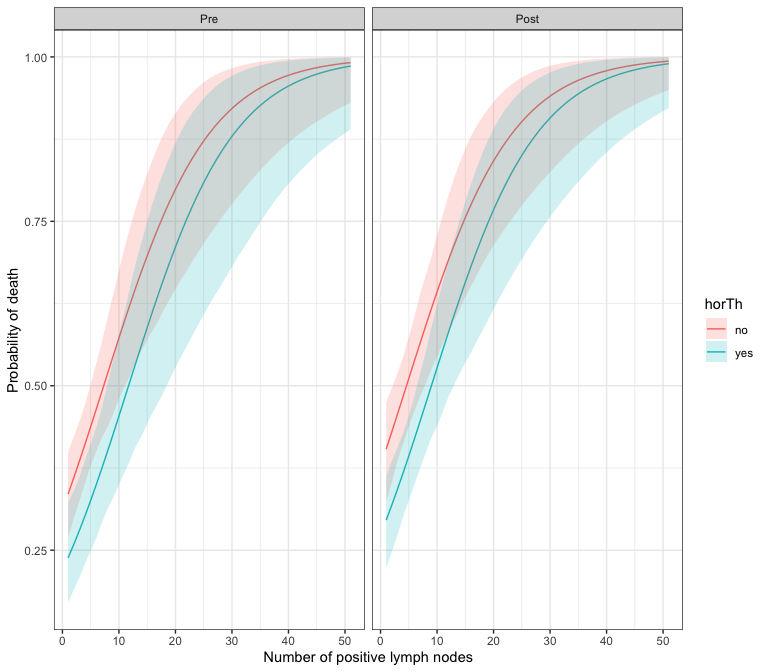# Bootstrap Simulation for model prediction

You can make a bootstrap simulation for model prediction. When writing bootPredict function and this vignette, I was inspired from package finalfit by Ewen Harrison. For example, you can predict survival after diagnosis of breast cancer.

library(autoReg)
library(dplyr)  # for use %>%
data(GBSG2,package="TH.data")
horTh age menostat tsize tgrade pnodes progrec estrec time cens
1    no  70     Post    21     II      3      48     66 1814    1
2   yes  56     Post    12     II      7      61     77 2018    1
3   yes  58     Post    35     II      9      52    271  712    1
4   yes  59     Post    17     II      4      60     29 1807    1
5    no  73     Post    35     II      1      26     65  772    1
6    no  32      Pre    57    III     24       0     13  448    1

Data GBGS2 in TH.data package is a data frame containing the observations from the German Breast Cancer Study Group 2. In this data, the survival status of patients is coded as 0 or 1 in the variable cens. Whether the patient receive the hormonal therapy or not is recorded as ‘no’ or ‘yes’ in variable horTh. The number of positive lymph nodes are recoded in pnodes. You can make a logistic regression model with the following R code.

GBSG2$cens.factor=factor(GBSG2$cens,labels=c("Alive","Died"))
fit=glm(cens.factor~horTh+pnodes+menostat,data=GBSG2,family="binomial")
summary(fit)

Call:
glm(formula = cens.factor ~ horTh + pnodes + menostat, family = "binomial",
data = GBSG2)

Coefficients:
Estimate Std. Error z value Pr(>|z|)
(Intercept)  -0.79237    0.15183  -5.219 1.80e-07 ***
horThyes     -0.47782    0.17578  -2.718  0.00656 **
pnodes        0.10853    0.01818   5.970 2.38e-09 ***
menostatPost  0.29375    0.16905   1.738  0.08228 .
---
Signif. codes:  0 '***' 0.001 '**' 0.01 '*' 0.05 '.' 0.1 ' ' 1

(Dispersion parameter for binomial family taken to be 1)

Null deviance: 939.68  on 685  degrees of freedom
Residual deviance: 887.36  on 682  degrees of freedom
AIC: 895.36

Number of Fisher Scoring iterations: 4

You can make a publication-ready table with the following R command.

autoReg(fit) %>% myft()

Dependent: cens.factor

Alive (N=387)

Died (N=299)

OR (multivariable)

horTh

no

235 (60.7%)

205 (68.6%)

yes

152 (39.3%)

94 (31.4%)

0.62 (0.44-0.88, p=.007)

pnodes

Mean ± SD

3.8 ± 4.6

6.5 ± 6.1

1.11 (1.08-1.16, p<.001)

menostat

Pre

171 (44.2%)

119 (39.8%)

Post

216 (55.8%)

180 (60.2%)

1.34 (0.96-1.87, p=.082)

You can draw a plot summarizing the model.

modelPlot(fit)For bootstrapping simulation, you can make new data with the following R code.

newdata=expand.grid(horTh=factor(c(1,2),labels=c("no","yes")),
pnodes=1:51,
menostat=factor(c(1,2),labels=c("Pre","Post")))

You can make a bootstrapping simulation with bootPredict() function. You can set the number of simulation by adjusting R argument.

df=bootPredict(fit,newdata,R=500)
horTh pnodes menostat  estimate     lower     upper
1    no      1      Pre 0.3354033 0.2692219 0.3999833
2   yes      1      Pre 0.2383652 0.1701567 0.3221579
3    no      2      Pre 0.3600105 0.2979548 0.4219831
4   yes      2      Pre 0.2586235 0.1900193 0.3398951
5    no      3      Pre 0.3853762 0.3245573 0.4454259
6   yes      3      Pre 0.2799707 0.2082833 0.3609657

With this result, you can draw a plot showing bootstrapping prediction of breast cancer.

library(ggplot2)
ggplot(df,aes(x=pnodes,y=estimate))+
geom_line(aes(color=horTh))+
geom_ribbon(aes(ymin=lower,ymax=upper,fill=horTh),alpha=0.2)+
facet_wrap(~menostat)+
theme_bw()+
labs(x="Number of positive lymph nodes", y="Probability of death")